Nuclear Science
Volume 1, Issue 1, December 2016, Pages: 18-23

Multipole Mixing Ratio, (E2/M1), and Electric Monopole Strength, (E0/E2), for γ-Transitions in 192Pt

Salah A. Eid1, Sohair M. Diab2

1Physics Department, Faculty of Engineering, Ain Shams University, Cairo, Egypt

2Physics Department, Faculty of Education, Ain Shams University, Cairo, Egypt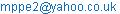(S. A. Eid)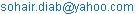(S. M. Diab)

Salah A. Eid, Sohair M. Diab. Multipole Mixing Ratio, (E2/M1), and Electric Monopole Strength, (E0/E2), for γ-Transitions in 192Pt. Nuclear Science. Vol. 1, No. 1, 2016, pp. 18-23. doi: 10.11648/j.ns.20160101.13

Received: October 29, 2016; Accepted: November 28, 2016; Published: January 10, 2017

Abstract: The low-lying collective states in 192Pt are investigated in the frame work of the interacting boson approximation model IBA-2. The contour plot of the potential energy surfaces, V(β,γ), shows an O(6) character to the nucleus. B(M1), B(E2), δ(E2/M1) and X(E0/E2) were calculated and reasonable agreement has obtained to the available experimental data. Also, sharp upper bending is observed at I+=10.

Keywords: Levels Energy, Transition Probability, B(M1), B(E2), Electric Monopole Strength, X(E0/E2) and Mixing Ratio δ(E2/M1)

1. Introduction

The low-lying states of 192Pt have been investigated by many authors experimentally and theoretically since they are lying in the transitional region between the end of the rare earth nuclei and the doubly closed shell of 208Pb.

The collective properties for the low-lying states of 192Pt have been investigated experimentally by means of Coulomb excitation using 3.3–4.8 MeV/nucleon 40Ca, 58Ni, 136Xe and 208Pb beams  and also by β- and electron capture decay of 192Ir [2-6]. The level structures of the shape transitional nuclei 190,192Pt have been studied by (α,xnγ) reactions on enriched Os targets. The measurements included γ-ray singles using Ge(Li) detectors, prompt and delayed γγ coincidences, half-life determinations in the range 1-500 ns, and γ-ray angular distributions. Detailed level scheme for 192Pt, incorporating much new spectroscopic information where they assigned spin, parity to levels and gamma rays multipolarity are reported. Acute back bending is observed at about spin 10 in the positive parity yarest sequences which is attributed to intersection of the ground bands .

Theoretically analytical solution for the Davydov-Chaban Hamiltonian  lead to simpler special potentials, levels energy and B(E2) for β and γ bands. Also, the calculated levels, J, π of ground, β and γ bands, B(E2), γ branching ratios, probability density contours in (β, γ) plane; deduced evidence of shape evolution and possible shape coexistence [8-11]. The calculated prolate and oblate levels, J of low-lying positive-parity states, quadrupole moment, quadrupole deformation parameter β2, contour diagrams of the ground state energies . The effective IBA Hamiltonian is used  to describe the low-lying spectra and electromagnetic properties of several series of even-even isotopes including Pt and Os. They reproduced some of 250 experimental energies with rms deviation of about 100 keV as well as transition probabilities. The sdg boson model has been used  and a satisfactory description of E2 and E4 properties is obtained for Pt and Os nuclei. The model has also predicted dynamic shape transitions from O(6) to SU(3) limits for these nuclei. The values of g-factor were evaluated  from transient field measurements and the IBA-2 has been used in measuring mass variations of g(21+).

The main purpose of the present study is:

(a) To see what kind of nuclei is 192Pt;

(b) To calculate levels energy, reduced transition probability of gamma ray transitions since very few values are available in literature and back bending;

(c)To calculate the multipole mixing ratio, δ (E2/M1), and

the electric monopole transitions, X(E0/E2).

2. The Interacting Boson Approximation Model (IBA-2)

2.1. Levels Energy

The IBA-2 model of Arima [16-18]was applied to the positive- parity of the low - lying states in 192Pt. The Hamiltonian employed for the present calculation is given by puddu  as:

H= ɛ (d x d) + ɛν (d x d)ν +V¶¶+Vνν +KQ.Qν+M¶ν  (1)

Where e, eυ are the proton and neutron effective charge energies respectively and they are assumed equal e = eυ =ε. The third and fourth term of Eq. (1) represent the proton-neutron interactions operator which is given by:

Vρρ=∑L=0,2,4 ½C(2L+1)½[(d x d) ρL.(d x d) ρL](0)     (2)

The fifth term is the quadrupole operator which is given by the usual expression (K is the strength of the proton and neutron bosons quadrupole interactions):

Qρ= [(s x d)+ (d x s)] ρ+ χ ρ (d x s) ρ             (3)

ρ= ¶ or υ

The sixth term is the Majorana operator and is given by

M¶ν=2∑K=1,3 ςK(dx d ν).(dx d ν)+ (ds ν-s d ν)2.

(ds ν -s d ν)                                   (4)

ρ= ¶ or υ

2.2. Reduced Transition Probabilities, B (E2)

The electric quadrupole transition operator employed in this study is defined as

T(E2) = e Q+ eυ Qυ                           (5)

where:

T(E2): Absolute transition probability of the electric quadrupole (E2) transition,

e and eυ: The proton and neutron effective charges, and

Qρ: The quadrupole operators which is the same as that in Eq.(3).

The reduced electric quadrupole transition rates between Ii→If states are given by

B(E2,Ii-If)=[˂ If ‖T(E2) ‖ Ii ˃]2/(2Ii+1)                   (6)

where

Ii: The initial state of the electric quadrupole transition, and

If: The final state of the electric quadrupole transition.

The proton and neutron boson numbers N and Nυ respectively can be treated as parameters. They are fixed to be half the number of valence fermions and counted from the nearest closed shell. The closed shells considered here in the present calculation are 82 and 126 for protons and neutrons respectively.

The effective charges e and eυ depend on the total number of bosons N, number of neutron bosons Nυ, number of proton bosons N and the experimental value of B(E2, 21+ → 01+), where

N=N+ Nυ                          (7)

The effective charges are calculated using Eq.(8) with the help of the experimental value of B(E2, 21+ → 01+), .

1/ N=[NB(E2,2+1 - 0+1)/(N+3)]½=e + N ν /N          (8)

3. Results and Discussion

3.1. The Potential Energy Surfaces

The energy surface, E(β, γ), for 192Pt nucleus as a function of the deformation parameters β and γ has been calculated using the following expression by Ginocchio :

E Nυ N¶ (β, γ) = < N Nυ ;βγ | Hnp | N Nυ; βγ >

= εd (Nυ+ N2(1+ β2)-1 + β2(1+β2)-2 { k N Nυ[4-(X¯ +Xυ¯)βcos3 γ + X¯ Xυ¯ β2] + Nυ (Nυ -1) (1/10 C0 + 1/7 C2) β2}   (9)

where

Xρ¯ = (2/7)0.5 Xρ ρ= ¶ or υ                         (10)

The contour plot of the potential energy surfaces is presented in figure 1, where the relation is between the potential energy surfaces and deformation parameter β which are shown at different values of γ. The value of γ varied between 0º and 60º. Also, figure 1 shows the same previous relation but only at γ=0º (prolate) and γ=60º (oblate). It is clear from the graphs that 192Pt is an O(6) nucleus where the deformation on the prolate and oblate sides are nearly equal. The energy ratios, Table 1., are supporting that assumption.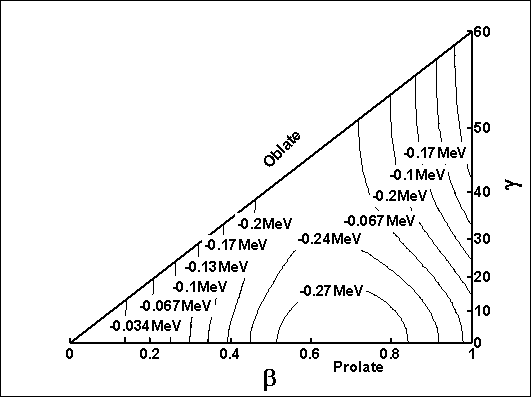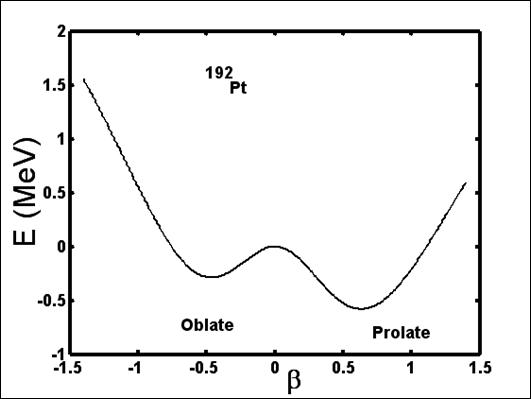Figure 1. Contour plot of the potential energy surfaces.

Table 1. Energy ratios for 192Pt.

 Quantity Exp*. IBA-2 SU(5) O(6) SU(3) E22+/E21+ 1.93 2.21 2.00 2.50 3.33 E41+/E21+ 2.48 2.61 2.00 2.50 3.33 E61+/E21+ 4.31 4.76 3.00 4.50 7.00 E42+/E21+ 3.80 4.00 3.00 4.50 7.00 E31+/E21+ 2.91 3.60 3.00 4.50 7.00 E81+/E21+ 6.38 7.37 4.00 7.00 12.00 E43+/E21+ 6.12 5.28 4.00 7.00 12.00

*Ref. 

3.2. Levels Energy

The calculated excitation energies of 192Pt are displayed in Figure 2. The agreement between the calculated and observed values by other authors is satisfactory.

The levels are reported experimentally  and classified into bands by Sakai . The Hamiltonian parameters of Eq. (1) used in the present calculation are:

ε = 0.180, K = -0.180, X = 0.180, Xυ = 0.100,

C =-0.280, -0.249, 0.030 C=-0.260, - 0.360, -0.090 all in MeV.

The computer codes NPBOS and NPBEM  of the IBA-2 have been used in calculating energy eigenvalues, wave functions and E2 transition matrix elements.Figure 2. Comparison between Exp.  and IBA levels energy.

3.3. Reduced Transition Probabilities, B (E2) and B(M1)

The proton and neutron effective charges e and eυ were determined using the experimental reduced transition probability B(E2,21+→01+)= 0.3750(6) e2b2 with the help of Hamilton  equations and the calculated values are:

e = 0.246 e. and eυ = 0.175 e.b

B(E2) values are calculated, then normalized to the experimental data of B(E2, 21+- 01+)=0.3750(6) and presented in Table 2. Unfortunately, few experimental data are available  where they compared to the present calculation.

To calculate M1 transition probability using IBA-2 model we take L=1 and B(M1) can be calculated for various transitions. The calculated values are presented in Table 2 and compared to the available experimental data.

Table 2. Transition probability ratios, B(E2) in (eb)2and B(M1) in (μN)2,

 Ii+ If+ B(E2) Exp*. B(E2) IBA Ii+ If+ B(E1) Exp*. B(E1) IBA 21 01 0.3750(6) 0.3999 11 01 —— 0.0083 02 21 —— 0.0661 11 02 —— 0.0013 22 01 0.0044 0.0042 22 21 0.0002 0.0006 22 02 —— 0.0002 23 21 —— 0.0054 22 21 0.4600 0.3882 24 21 —— 0.0022 23 21 —— 0.0010 31 21 0.0002 0.0002 24 21 —— 0.0007 31 22 0.0005 0.0009 23 22 —— 0.0078 31 41 0.0002 0.0008 24 22 —— 0.0017 42 31 —— 0.0000 31 21 0.0044 0.0043 42 41 —— 0.0014 31 22 0.6711 0.4980 42 32 —— 0.0003 41 21 0.5860 0.5541 42 33 —— 0.0046 41 22 —— 0.0043 24 22 —— 0.0017 42 21 —— 0.0013 24 23 —— 0.0150 31 41 0.2500 0.1728 32 21 —— 0.0089 42 41 —— 0.2317 32 22 —— 0.0002 42 22 —— 0.3074 32 23 —— 0.1072

* Ref 

3.4. Mixing Ratios δ(E2/M1)

To calculate the mixing ratios for γ - transitions we need first to calculate the gyromagnetic g- factors which is g for proton and gν for neutron. The relation between g – factors and magnetic moment μ (21+) is:

μ (21+) = 2g (21+)                              (11)

and

g = g [N/(N+Nν)] + gν [Nν / (N+Nν)]   (12)

Then, M1-E2 mixing parameters  is given by:

δ(E2/M1)= 0.835 E γ Δ(E2/M1)                (13)

where

Δ(E2/M1) = ˂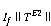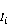˃/ ˂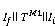˃     (14)

and

˂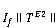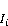˃ = e Q + eυ Qυ

= [(2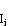+1)B(E2,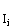-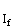)]½,                   (15)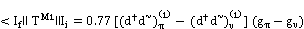(16)

Table 3. Multipole mixing ratios δ(E2/M1) in (eb/ μN).

 Ii+ If+ Eγ δexp.* IBA-2. 22 21 0.295 10.0±32 9.85 23 21 1.122 ---- 0.91 31 21 0.604 4.48±5 6.46 31 22 0.309 7.42±4 14.11 31 41 0.136 3.30 10.50 42 31 0.280 5.40 171.05 42 41 0.416 2.90 4.63

*Ref. 

The sign of δ(E2/M1) is given from the sign of the matrix elements used in the calculations. The calculated values are compared to the experimental data which show reasonable agreement and displayed in Table 3

3.5. Strength of the Electric Monopole Transitions, Xif'f (E0/E2)

The electric monopole transitions, E0, are normally occurring between two states of the same spin and parity by transferring energy and zero unit of angular momentum. It is a pure penetration effect where it caused by the electromagnetic interaction between the nuclear charge and the atomic electron which penetrates the nucleus. The strength of the electric monopole transition Xif'f (E0/E2)  can be determined by the relation:

Xif ′f (E0/E2) = B(E0, Ii – If) / B(E2, Ii – I'f)       (17)

Where

Ii = If = 0, I'f =2 or Ii = If ≠ 0, If = I'f

Xif′f (E0/E2 = 2.54x109 A3/4 [ E5γ MeV/ ΩKL]x α (E2) x

[Te(E0,Ii–If)/Te(E2,Ii–I'f)]                     (18)

A: Mass number;

Ii: Spin of the initial state where E0 and E2 transitions are

depopulating it;

If: Spin of the ﬁnal state of E0 transition;

I′ f:Spin of the ﬁnal state of E2 transition;

Eγ: Gamma ray energy;

KL:Electronic factor for K,L shells ;

α(E2): Conversion coecient of the E2 transition;

Te (E0, Ii − If): absolute transition probability of the E0 transition

between Ii and If states, and

Te (E2, Ii − I′ f): Absolute transition probability of the E2 transition between Ii and I′ f states.

The E0 strength can be considered as the ratio between the reduced transition probability of competing E0 and electric quadrupole, E2, transitions de-populating the same level. The calculated values are presented in Table 4 with the available experimental data.

Table 4. Calculated Xif ′ f (E0/E2).

 Ii+ If+ Xif'f (E0/E2) Exp* IBA-2 02 01 ------ 0.0100 03 01 ------ 0.5450 03 02 ------ 0.0007 04 01 ------ 0.0164 04 02 ------ 0.0050 04 03 ------ 0.0389 22 21 0.016±0.014 0.0006 23 21 ------ 0.9107 24 21 ------ 0.2727 23 22 ------ 0.0100 24 22 ------ 0.2857 24 23 ------ 0.0572

3.6. Back Bending

To study back bending we need a relation between the moment of inertia I and energy parameters ħω where we used Eq's (19, 20):

2J/ħ2=[ (4I-2) /ΔE(I→I-2)]                   (19)

(ħω)2= (I2-I+1)[ ΔE(I→I-2)/(2I-1)]               (20)

The relation between 2J/ħ2 and I is given in Figure 3 and sharp upper bending at I+=10 is observed which is due to ground state band crossing.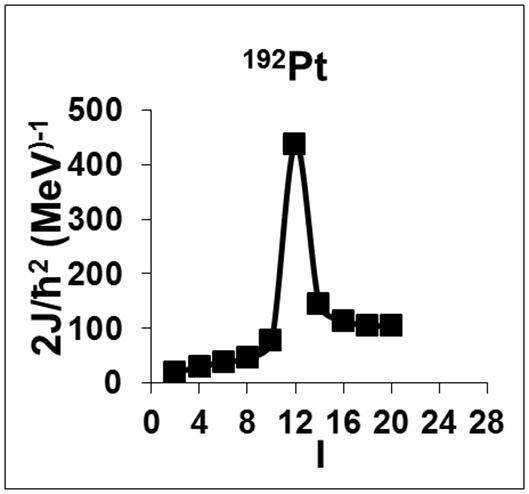Figure 3. Angular momentum I as a function of 2J/ħ2.

3.7. The Staggering

The presence of the odd-even parity states has encouraged us to study staggering effect for 192Pt. Staggering pattern between the (+ve) parity ground state and the (–ve) parity octupole band states have been calculated, ΔI=1, using staggering function equations (21, 22) with the help of the experimental data.

Stag.(I)=6 ΔE(I) - 4ΔE(I-1) - 4 ΔE(I+1)+ ΔE(I+2)+ ΔE(I-2)  (21)

ΔE(I) = E(I+1) - E(I)                     (22)

The calculated staggering pattern is presented in figure 4. We can see the beat pattern which indicate an interaction between the ground state and the octupole band states.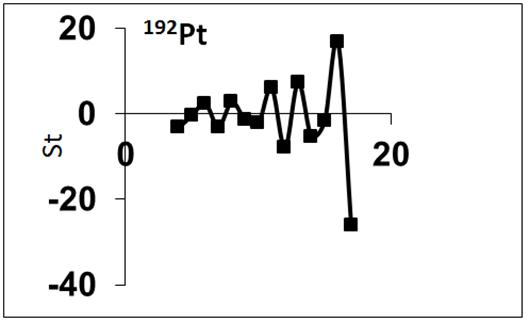Figure 4. Staggering pattern for 192Pt.

5. Conclusion

1. The contour plots of the potential energy surfaces, V(β, γ), for 192-202Pt presented in Figure 5. show that 192Pt is an O(6) nucleus and that is supported by γ – rays energy ratios, Table 1.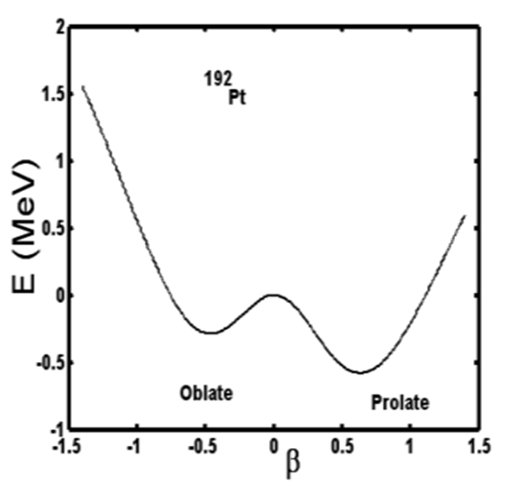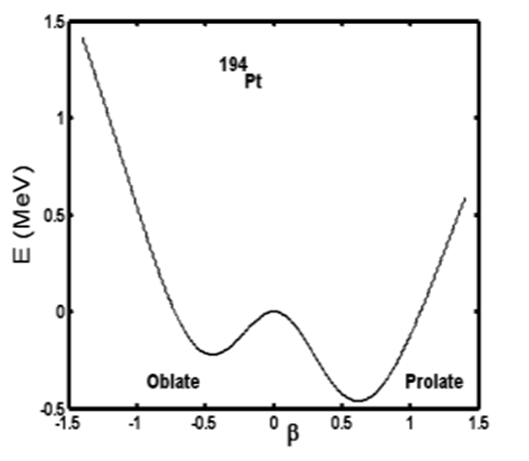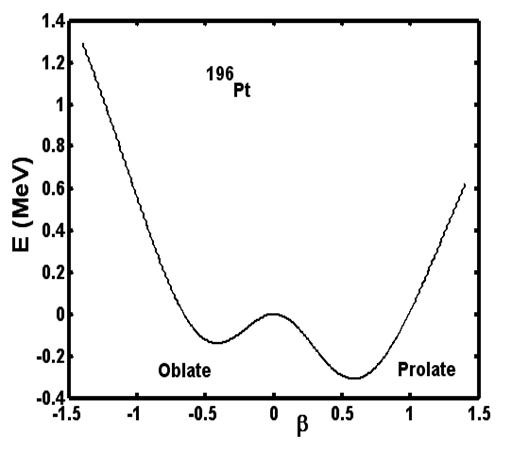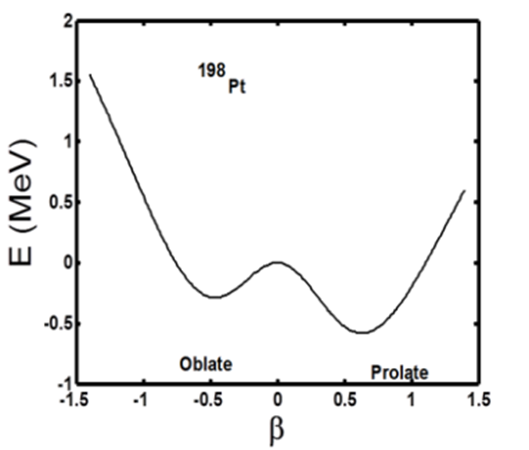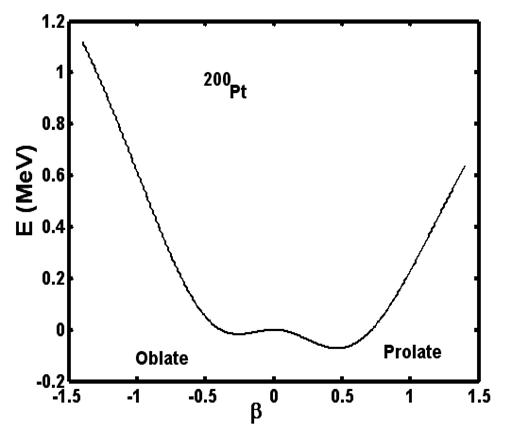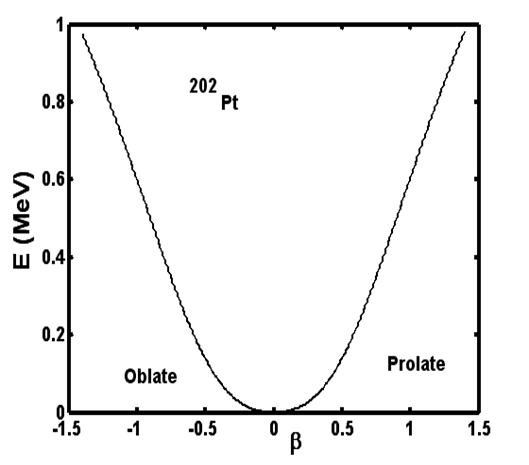Figure 5. Contour plot of the potential energy surfaces for 192-202Pt.

2. The levels energy for 192Pt were calculated using IBA-2 model. The agreement between the calculated and experimental values are fairly good for the low – lying states and slightly poor for high spin states which may be due to band crossing.

3. The transition probability of gamma transitions were calculated and normalized to B(E2,21+→01+)= 0.3750(6) e2b2. The agreement between the calculated and experimental values are good.

4. The multipolarity of γ- ray transitions and the electric monopole strength are calculated. They show reasonable agreement to the available measured experimental values.

References

1. W. C. Y, Cline, D. Czosnyka,T. Backlin, A. Baktash,C.iamond, R. M, Dracoulis, G. D, Hasselgren, L. Kluge, H. Kotlinski, B. Leigh, J. R, Newton, J. O, Phillips, W. R. Sie, S. H. Srebrny, F. S. Stephens, "Quadrupole collectivity andshapes of Os and Pt nuclei "; Nucl. Phys. A607, 178, (1996)
2. H. EL Samman, W. Arafa, S. Eid, M. Abd Elwihab andF. Sayd,; "On The Level Structure of l92 Pt and I92 Os Nuclei"Nucl. Science J. 32, 335, (1995)
3. L. Yabo, H. Dailing, S. Hueibin and D. Zhaozhong; "studieson the low-lying levels in 192Pt populated in 192Ir decay"Z.Phys. A329, 307, (1988)
4. D. Mehta, S. Singh, M.L. Garg, N. Singh, T.S. Cheemaand P.N. Trehan,; " Study of the radioactive decay of 192Ir"Z.Phys. A328, 31,(1987)
5. E. Eid, and M. Stewart.; "studies on the low-lying levels in192Pt and 192Os" Z. Phys. A320, 495, (1985)
6. J. C. Cunnane, M. piiparinen, and P. J. Daly; "High-spin levelstructure of 190Pt and 192Pt", Phys. Rev. C13, 2197, (1976)
7. P. Buganu and R. Budaca;"Analytical solution for theDavydov- Chaban Hamiltonian with a sextic potential forγ=30", Phys. Rev. C 91, 014306, (2015)
8. P. Buganu and A. A. Raduta; "Energy Spectra, E2 TransitionProbabilities and Shape Deformations for the Even-EvenIsotopes 180-196Pt", Rom. J. Phys. 60, 161, (2015)
9. J. E. García-Ramos, K. Heyde, L. M. Robledo, and R. Rodríguez-Guzmán;"Shape evolution and shape coexistence inPt isotopes: Comparing interacting boson model configurationmixing and Gogny mean-field energy surfaces", Phys. Rev. C89, 034313, (2014)
10. A. A. Raduta and P. Buganu; " Description of the isotope chain180−196Pt within several solvable approaches", Phys. Rev. C88, 064328, (2013)
11. H.G. Gane v; "Transition probabilities in the U (3,3) limit of thesymplectic interacting vector boson model", Phys. Rev. C 86,054311, (2012)
12. Yu Zhang, Feng Pan, Yu-Xin Liu, Yan-An Luo, and J. P.Draayer;"Analytically solvable prolate-oblate shape phasetransitional description within the SU (3) limit of the interactingboson model",Phys. Rev. C 85, 064312, (2012)
13. A. Gomez, O. Castanos, and A. Frank, "Description of somechains of isotopes and isotones in the interacting-bosonapproximation "; Nucl. Phys. A589, 267, (1995)
14. V.S. Lac, and S. Kuyucak,;"Description of transitionalnuclei in sdg boson model" Nucl. Phys. A 539, 418, (1992)
15. A. E. Stuchbery, G. J Lampard, and H. H. Bolotin;"Transientfield measurements of g-factor in Pt; g (21+) systematics intransitional W, Os, Pt nuclei" Nucl. Phys. A528, 447, (1991)
16. A. Arima and F. Iacello.; "Interacting boson model of collectivestates: The \$O(6)\$ limit.", Ann. Phys., 123, 468, (1979) ", (N.Y.), Ann. phys. 123, 468, (1979)
17. A. Arima and F. Iacello; Interacting boson model of collectivestates: The vibrational limit."The interacting boson model",Ann. phys., (N.Y.), 99, 253, (1976)
18. A. Arima and F. Iacello.; "Interacting boson model of collectivestates: The rotational limit", Ann. phys.,(N.Y.), 111, 201, (1978)
19. G. Puddu and O. Scholten, "Collective Quadrupole States ofXe, Ba and Ce In the Interacting Boson Approximation model,"Nucl. Phys. A 348, 109, 124, (1980)
20. W. D. Hamilton, P. Irback and J. P. Elliott, "Mixed-SymmetryInteracting Boson Model In the Nuclei 140Ba, 142Ce and 144Ndwith N= 84, " Phys. Rev. Lett. 53, 2469, 2472, (1984)
21. J. N. Ginocchio and M. W. Kirson, "An intrinsic state for theinteracting boson model and its relationship to the Bohr-Mottelson model," Nucl. Phys. A350, 31, (1980)
22. C. M. Baglin, "ADOPTED LEVELS, GAMMAS for 192Pt", Nucl. Data Sheets; 113, 1871, (2012).
23. M. Sakai, "Quasi-bands in even - even nuclei," Atom. Nucl.Data. Tab. 31, 399, (1984).
24. T. Otsuka, Program Package NPBOS K. V. I- Report No. 63 (1979)
25. M. A. Kadhim, A. S. Mohammed, A. A. Shareefi, A. R. Subber, "δ(E2/M1) and X(E0/E2) ratios for 192-202Pt isotopes by using theproton and neutron interacting boson model (IBM-2)",InternationalJournal of Physics., 3 (3), 120, (2015)
26. S. Raman, C. W. Nestor. and P. Tikkanen.; "Transitionprobability from the ground to the first – excited 2+ state ofeven-even nuclides", Atom. Data and Nucl. Data Tab. 78, 1, (2001)
27. J. O. Rasmussen, "Theory of E0 transitions of spheroidalnuclei," Nucl. phys.19, (1960)
28. A. D. Bell, C. E. Aveldo, M. G. Davidson and J. P. Davidson, "Table of E0 Conversion Probability Electronic Factors," Can. J. Phys. 48, 2542, 2570, (1970)

 Contents 1. 2. 2.1. 2.2. 3. 3.1. 3.2. 3.3. 3.4. 3.5. 3.6. 3.7. 5.
Article ToolsAbstractPDF(472K)